## Continuity

A function f( x) is said to be continuous at a point ( c, f( c)) if each of the following conditions is satisfied:Geometrically, this means that there is no gap, split, or missing point for f( x) at c and that a pencil could be moved along the graph of f( x) through ( c, f( c)) without lifting it off the graph. A function is said to be continuous at ( c, f( c)) from the right if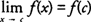and continuous at ( c, f( c)) from the left if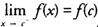. Many of our familiar functions such as linear, quadratic and other polynomial functions, rational functions, and the trigonometric functions are continuous at each point in their domain.

A special function that is often used to illustrate one‐sided limits is the greatest integer function. The greatest integer function, [ x], is defined to be the largest integer less than or equal to x (see Figure 1).Figure 1 The graph of the greatest integer function y = [ x].

Some values of [ x] for specific x values are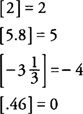The greatest integer function is continuous at any integer n from the right only because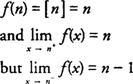hence,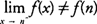and f( x) is not continuous at n from the left. Note that the greatest integer function is continuous from the right and from the left at any noninteger value of x.

Example 1: Discuss the continuity of f( x) = 2 x + 3 at x = −4.

When the definition of continuity is applied to f( x) at x = −4, you find thathence, f is continous at x = −4.

Example 2: Discuss the continuity of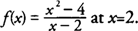When the definition of continuity is applied to f( x) at x = 2, you find that f(2) does not exist; hence, f is not continuous (discontinuous) at x = 2.

Example 3: Discuss the continuity of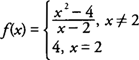When the definition of continuity is applied to f( x) at x = 2, you find that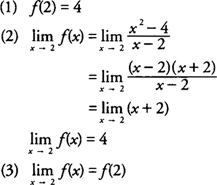hence, f is continous at x = 2.

Example 4: Discuss the continuity of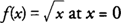.

When the definition of continuity is applied to f( x) at x = 0, you find thathence, f is continuous at x = 0 from the right only.

Example 5: Discuss the continuity ofWhen the definition of continuity is applied to f( x) at x = −3, you find thatMany theorems in calculus require that functions be continuous on intervals of real numbers. A function f( x) is said to be continuous on an open interval ( a, b) if f is continuous at each point c ∈ ( a, b). A function f( x) is said to be continuous on a closed interval [ a, b] if f is continuous at each point c ∈ ( a, b) and if f is continuous at a from the right and continuous at b from the left.

Example 6:

a. f( x) = 2 x + 3 is continuous on (−∞,+∞) because f is continuous at every point c ∈ (−∞,+∞).

b. f( x) = ( x − 3)/( x + 4) is continuous on (−∞,−4) and (−4,+∞) because f is continuous at every point c ∈ (−∞,−4) and c ∈ (−4,+∞)

c. f( x) = ( x − 3)/( x + 4) is not continuous on (−∞,−4] or [−4,+∞) because f is not continuous on −4 from the left or from the right.

d.is continuous on [0, +∞) because f is continuous at every point c ∈ (0,+∞) and is continuous at 0 from the right.

e. f( x) = cos x is continuous on (−∞,+∞) because f is continuous at every point c ∈ (−∞,+∞).

f. f( x) = tan x is continuous on (0,π/2) because f is continuous at every point c ∈ (0,π/2).

g. f( x) = tan x is not continuous on [0,π/2] because f is not continuous at π/2 from the left.

h. f( x) = tan x is continuous on [0,π/2) because f is continuous at every point c ∈ (0,π/2) and is continuous at 0 from the right.

i. f( x) = 2 x/( x 2 + 5) is continuous on (−∞,+∞) because f is continuous at every point c ∈ (−∞,+∞).

j. f( x) = | x − 2|/( x − 2) is continuous on (−∞,2) and (2,+∞) because f is continuous at every point c ∈ (−∞,2) and c ∈ (2,+∞).

k. f( x) = | x − 2|/( x − 2) is not continuous on (−∞,2] or [2,+∞) because f is not continuous at 2 from the left or from the right.